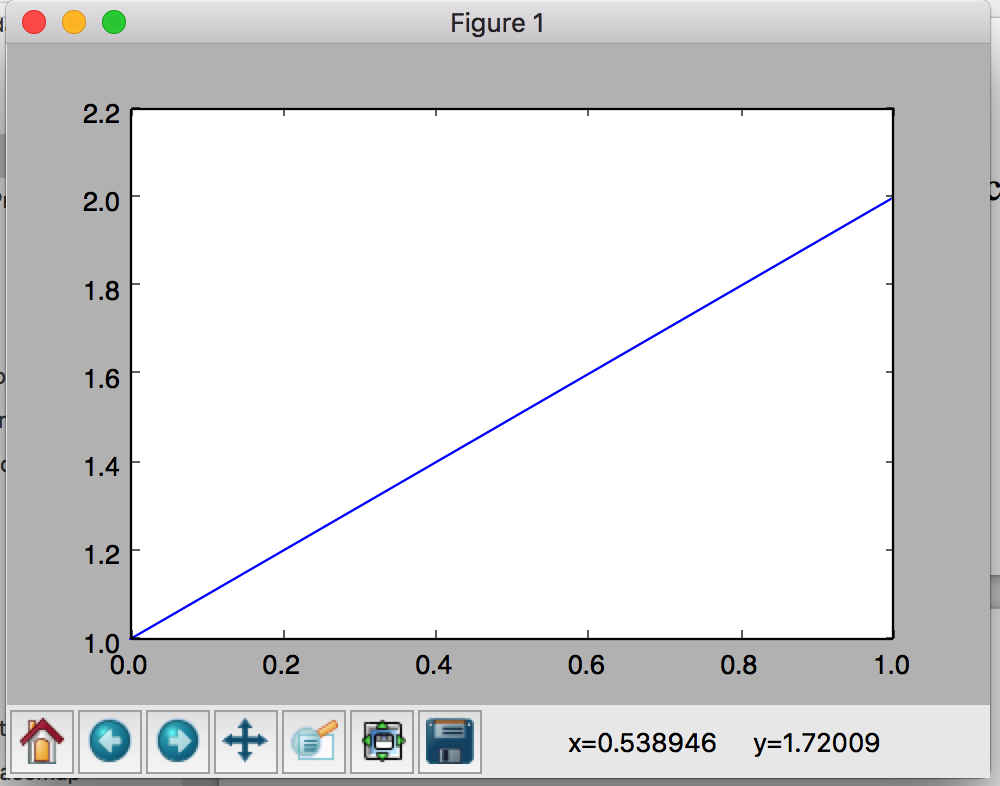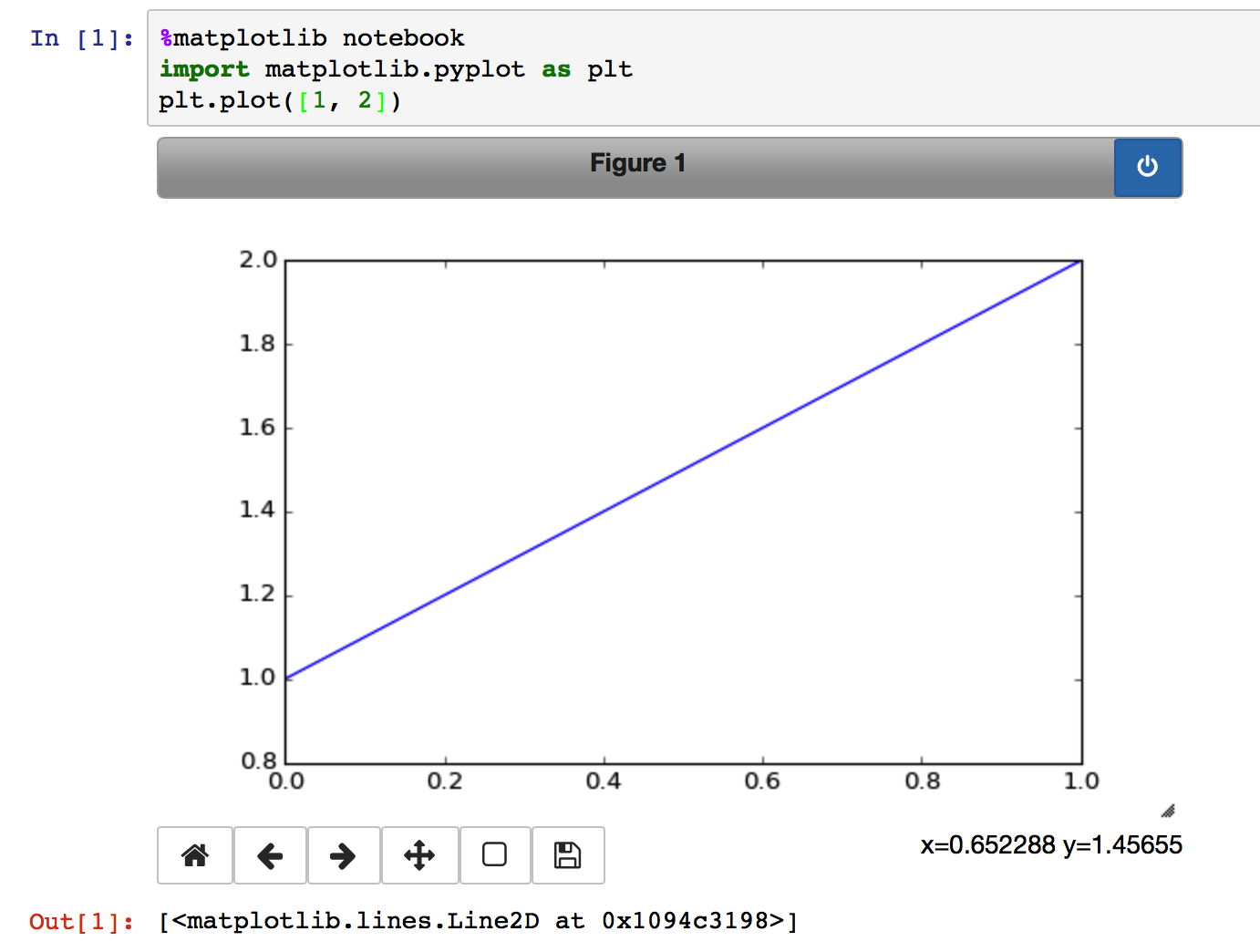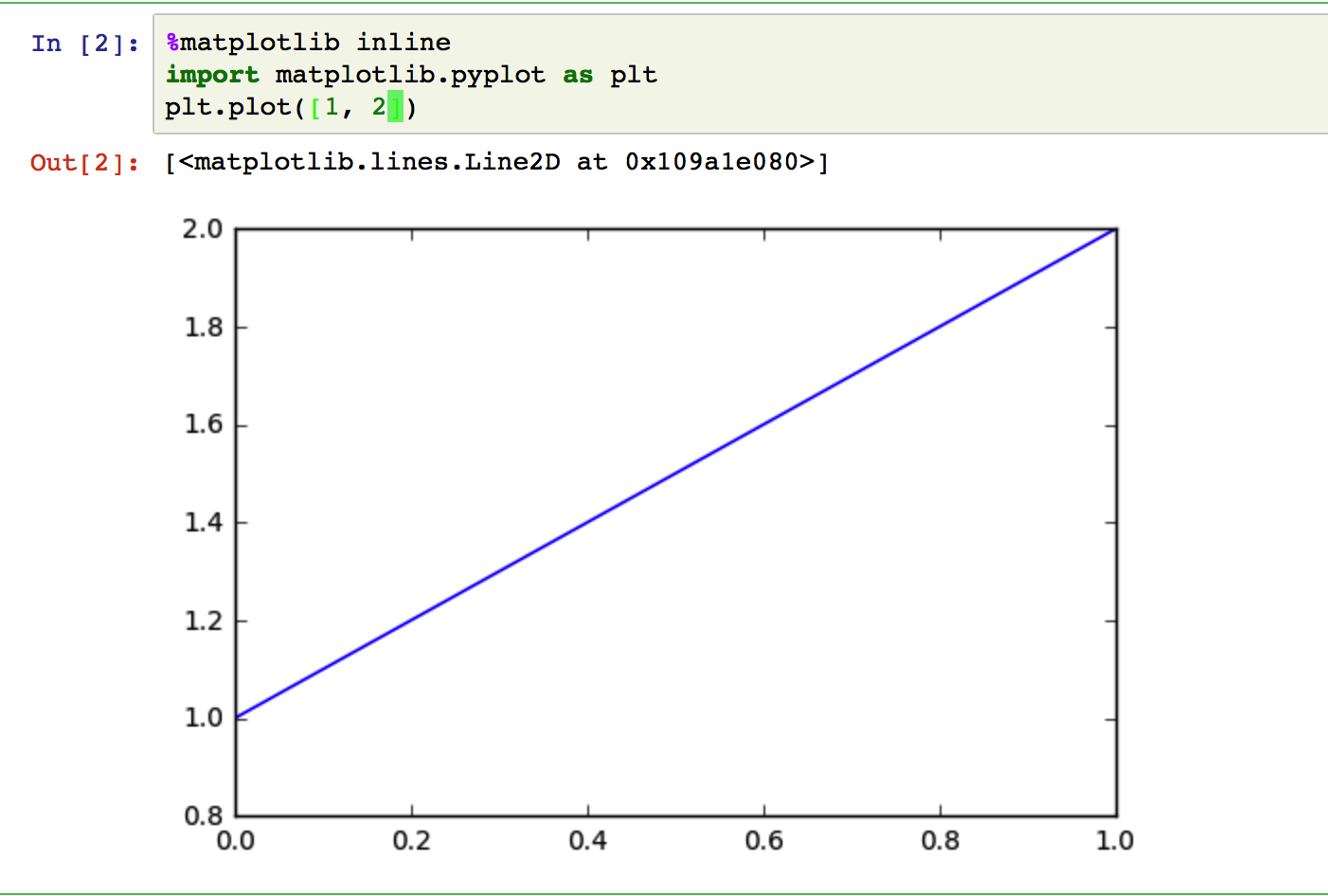# Onway

posts - 61, comments - 56, trackbacks - 0, articles - 34

python中用于数据库可视化的2D绘图库。

http://matplotlib.org/users/installing.html

1，非交互模式

python shell里面执行

import matplotlib.pyplot as plt
plt.plot()
plt.show()2，交互模式

matplotlib.is_interactive()可以查看当前是否在交互模式；

matplotlib.pyplot.ion()用于打开交互模式；

matplotlib.pyplot.ioff()用于关闭交互模式；

import matplotlib.pyplot as plt
plt.ion()
plt.plot()

plt.plot()

3，ipython的magic command

1，%matplotlib

2，％matplotlib notebook3，%matplotlib inlinehttp://matplotlib.org/faq/usage_faq.html#parts-of-a-figure

1matlab风格接口

plt.plot()
plt.plot()

2，面向对象接口

fig, ax = plt.subplots()
ax.plot()
ax.plot()

3，差别

http://matplotlib.org/gallery.html

http://matplotlib.org/api/index.html